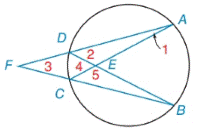Chapter 6.2, Problem 1E### Elementary Geometry for College St...

6th Edition
Daniel C. Alexander + 1 other
ISBN: 9781285195698

#### Solutions

Chapter
Section### Elementary Geometry for College St...

6th Edition
Daniel C. Alexander + 1 other
ISBN: 9781285195698
Textbook Problem
33 views

# Given: m A B ⌢ = 92 ° m D A ⌢ = 114 ° m B C ⌢ = 138 ° Find: a) m ∠ 1( ∠ D A C ) b) m ∠ 2 ( ∠ A D B ) c) m ∠ 3 ( ∠ A F B ) d) m ∠ 4 ( ∠ D E C ) e) m ∠ 5 ( ∠ C E B )To determine

(a)

To find:

m1 (DAC) when mAB=92°, mDA=114° and mBC=138°.

Explanation

Corollary:

The measure of an angle formed by a tangent and a chord drawn to the point of tangency is one-half the measure of the intercepted arc.

Calculation:

m1 (DAC).

mAB=92°, mDA=114° and mBC=138°.

By corollary,

m1=12(mCD)

mAB+mBC+mCD

To determine

(b)

To find:

m2 (ADB) when mAB=92°, mDA=114° and mBC=138°.

To determine

(c)

To find:

m3 (AFB) when mAB=92°, mDA=114° and mBC=138°

To determine

(d)

To find:

m4 (DEC) when mAB=92°, mDA=114° and mBC=138°.

To determine

(e)

To find:

m5 (CEB) when mAB=92°, mDA=114° and mBC=138°.

### Still sussing out bartleby?

Check out a sample textbook solution.

See a sample solution

#### The Solution to Your Study Problems

Bartleby provides explanations to thousands of textbook problems written by our experts, many with advanced degrees!

Get Started

#### Find more solutions based on key concepts